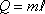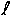Heat Q = mass m times latent heatIf you add heat to a liquid that is at its boiling point (boiling temperature) the liquid changes to a gas. The amount of heat that it takes to convert mass m of the liquid to a gas is equal to the mass m times the latent heat of vaporization. So the latent heat of vaporization is the heat per mass that it takes to convert the liquid to gas.

If you add heat to a solid that is at its melting point (melting temperature) the liquid changes to a gas. The amount of heat that it takes to convert mass m of the solid to a liquid is equal to the mass m times the latent heat of melting. So the latent heat of melting is the heat per mass that it takes to convert the solid to a liquid.